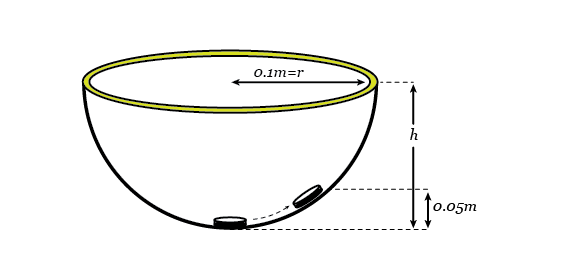# What goes up sometimes stays there

Accelerometers are commonly found in mobile game devices - they measure the motion of the device which can be used to interact with a game. A simple accelerometer can be constructed by placing a small coin in a hemispherical bowl. If you are not accelerating, the coin rests at the bottom of the bowl. If, however, you are accelerating horizontally the coin will slide up the side until it stops at some height and stays there.

If the bowl has a radius of 0.1 m and the coin comes to rest at a vertical height of 0.05 m above the bottom of the bowl, what is the magnitude of your acceleration in $m/s^2$?Details and assumptions

• Neglect any friction between the sides of the bowl and the coin.
• The acceleration due to gravity is $-9.8~m/s^2$.
×